Скачать презентацию 5 -8 Scale Drawings and Scale Models 7

d52ab5ea3d50ac389857f8836ac2eaf2.ppt

• Количество слайдов: 13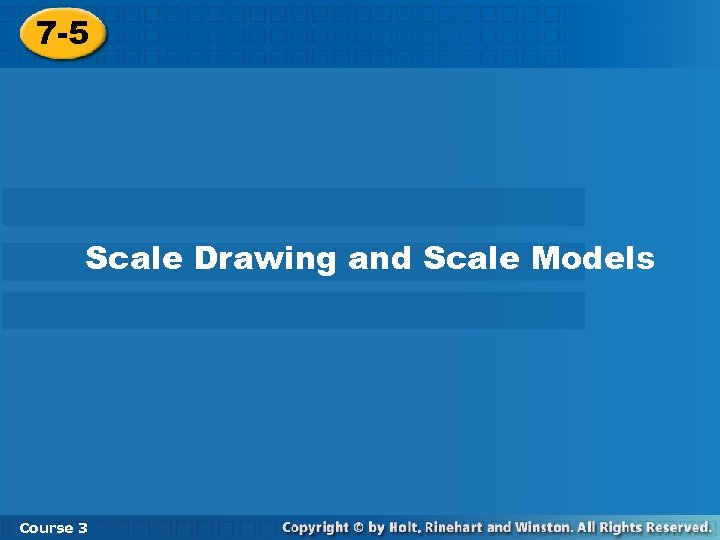5 -8 Scale Drawings and Scale Models 7 -5 Scale Drawing and Scale Models Course 3 3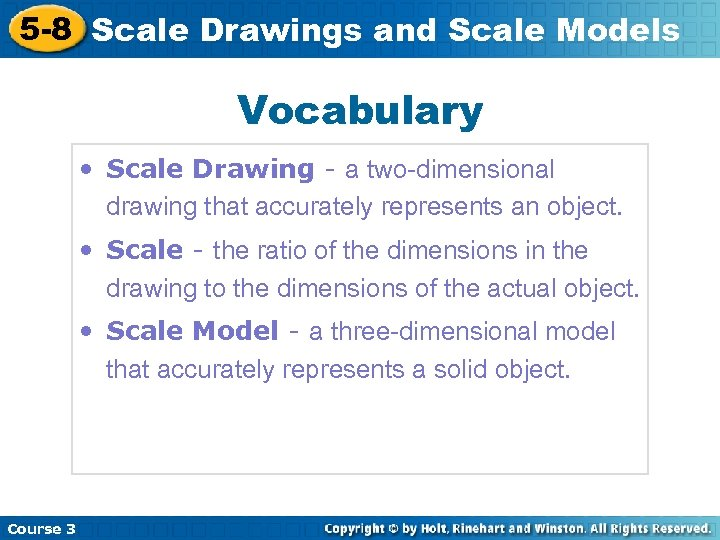5 -8 Scale Drawings and Scale Models Vocabulary • Scale Drawing - a two-dimensional drawing that accurately represents an object. • Scale - the ratio of the dimensions in the drawing to the dimensions of the actual object. • Scale Model - a three-dimensional model that accurately represents a solid object. Course 3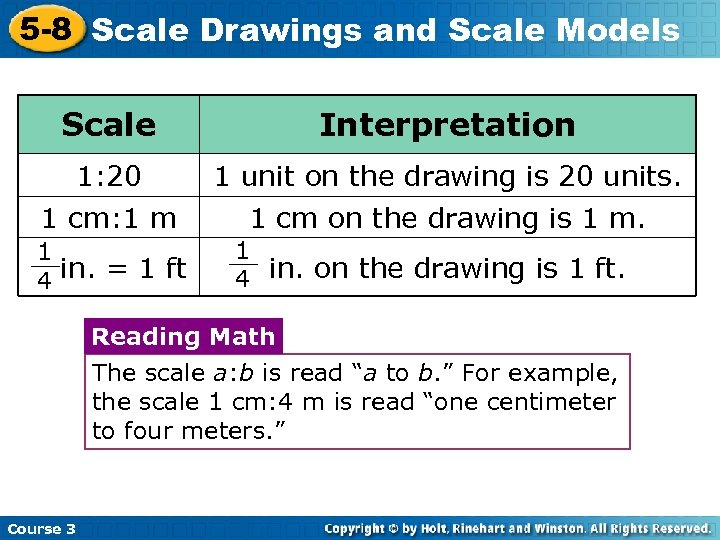5 -8 Scale Drawings and Scale Models Scale Interpretation 1: 20 1 unit on the drawing is 20 units. 1 cm: 1 m 1 cm on the drawing is 1 m. 1 4 in. = 1 ft 1 4 in. on the drawing is 1 ft. Reading Math The scale a: b is read “a to b. ” For example, the scale 1 cm: 4 m is read “one centimeter to four meters. ” Course 3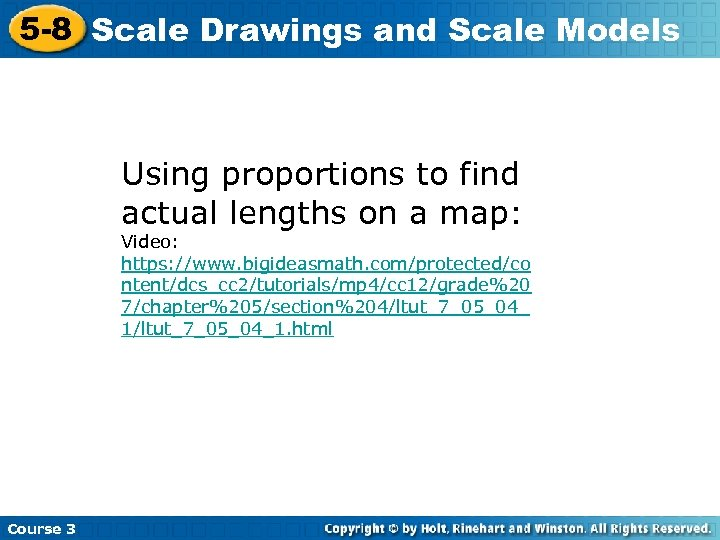5 -8 Scale Drawings and Scale Models Using proportions to find actual lengths on a map: Video: https: //www. bigideasmath. com/protected/co ntent/dcs_cc 2/tutorials/mp 4/cc 12/grade%20 7/chapter%205/section%204/ltut_7_05_04_ 1/ltut_7_05_04_1. html Course 3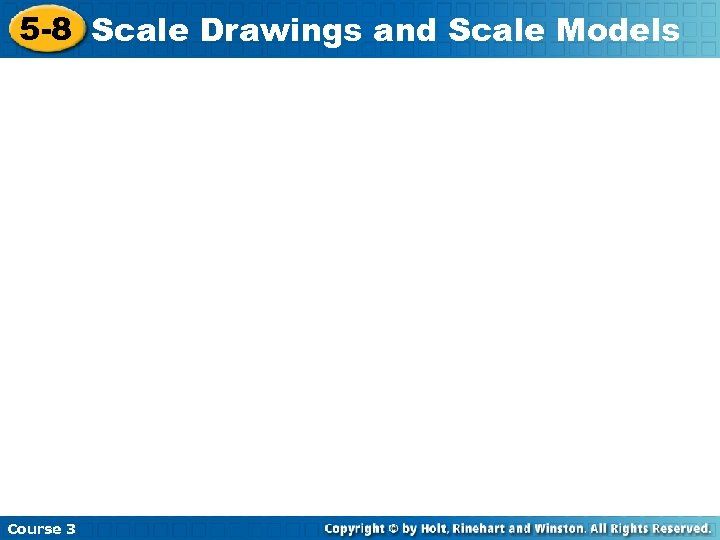5 -8 Scale Drawings and Scale Models Course 3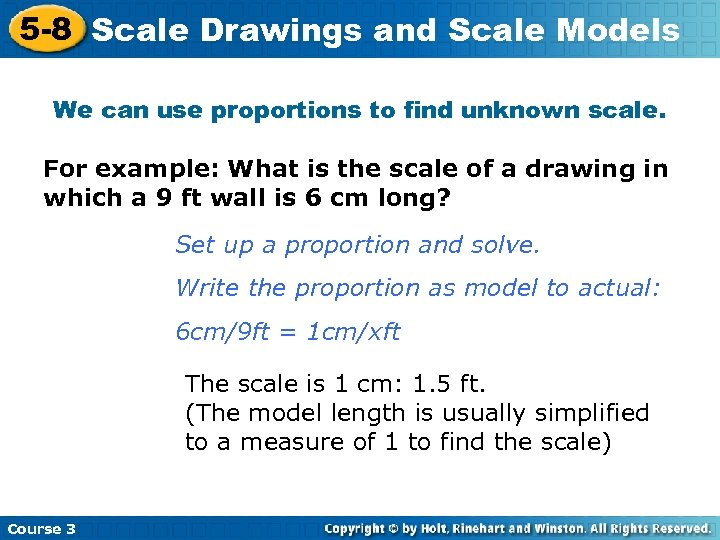5 -8 Scale Drawings and Scale Models We can use proportions to find unknown scale. For example: What is the scale of a drawing in which a 9 ft wall is 6 cm long? Set up a proportion and solve. Write the proportion as model to actual: 6 cm/9 ft = 1 cm/xft The scale is 1 cm: 1. 5 ft. (The model length is usually simplified to a measure of 1 to find the scale) Course 3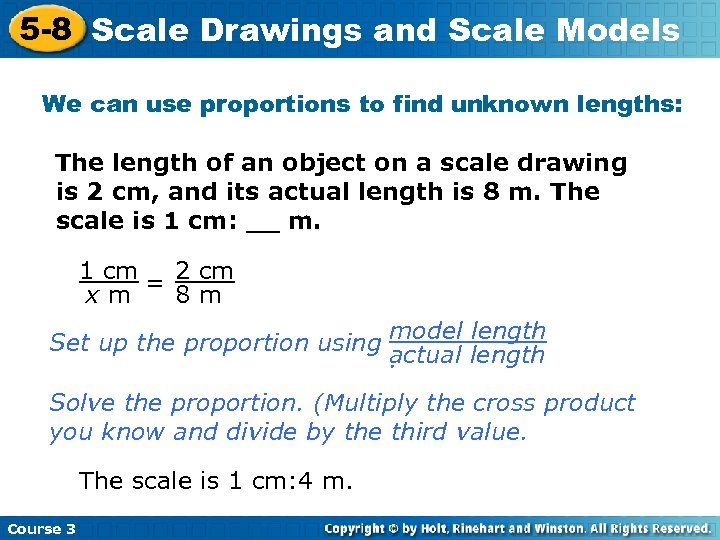5 -8 Scale Drawings and Scale Models We can use proportions to find unknown lengths: A. The length of an object on a scale drawing is 2 cm, and its actual length is 8 m. The scale is 1 cm: __ m. 1 cm 2 cm = 8 m xm length Set up the proportion using model length actual. Solve the proportion. (Multiply the cross product you know and divide by the third value. The scale is 1 cm: 4 m. Course 3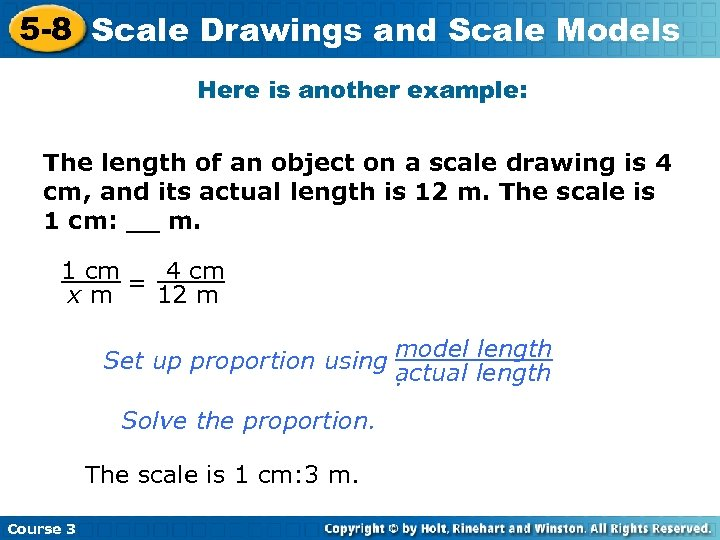5 -8 Scale Drawings and Scale Models Here is another example: The length of an object on a scale drawing is 4 cm, and its actual length is 12 m. The scale is 1 cm: __ m. 1 cm 4 cm = 12 m xm length Set up proportion using model length actual. Solve the proportion. The scale is 1 cm: 3 m. Course 3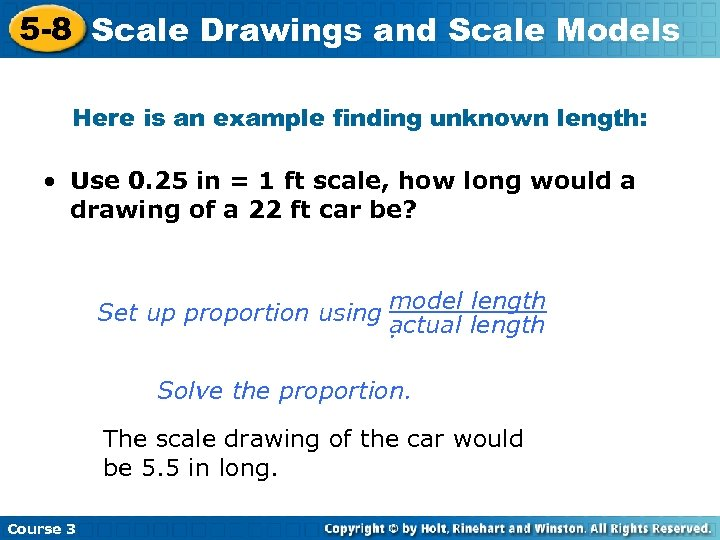5 -8 Scale Drawings and Scale Models Here is an example finding unknown length: • Use 0. 25 in = 1 ft scale, how long would a drawing of a 22 ft car be? length Set up proportion using model length actual. Solve the proportion. The scale drawing of the car would be 5. 5 in long. Course 35 -8 Scale Drawings and Scale Models Your turn: Finding Unknown Dimensions Given Scale Factors A model of a 27 ft tall house was made using a scale of 2 in: 3 ft. What is the height of the model? Set up a proportion and solve The height of the model is 18 in. Course 3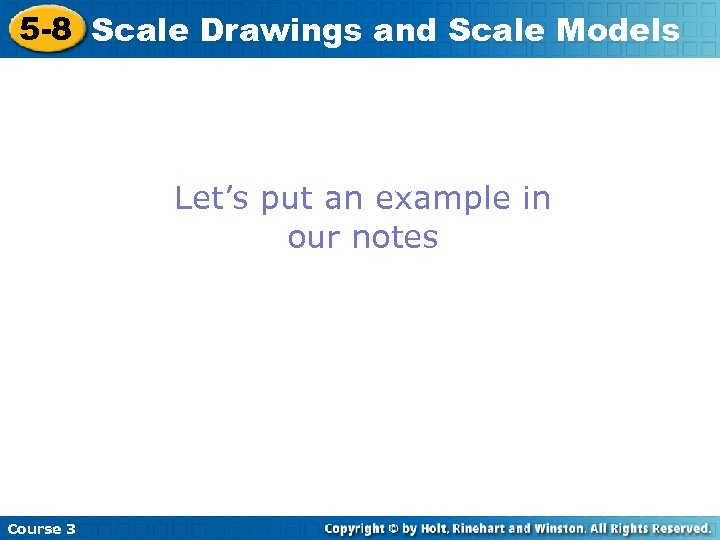5 -8 Scale Drawings and Scale Models Let’s put an example in our notes Course 3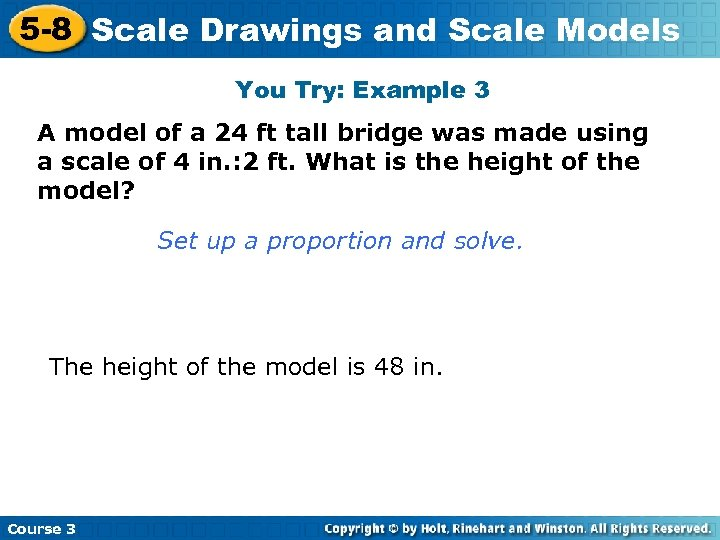5 -8 Scale Drawings and Scale Models You Try: Example 3 A model of a 24 ft tall bridge was made using a scale of 4 in. : 2 ft. What is the height of the model? Set up a proportion and solve. The height of the model is 48 in. Course 3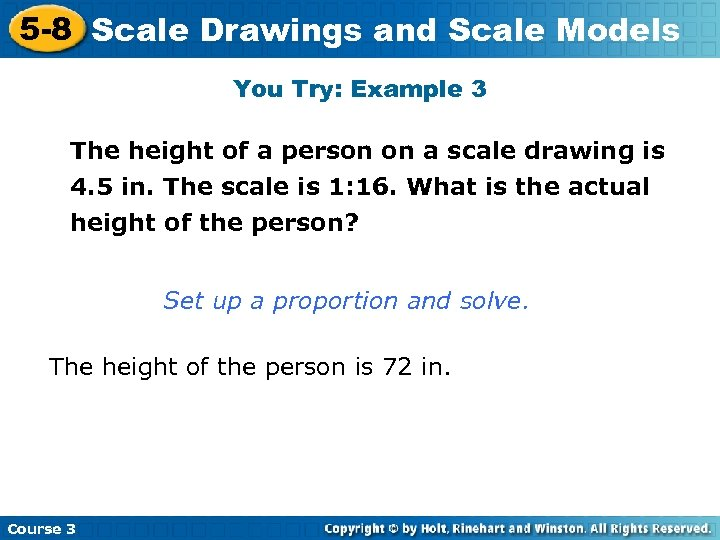5 -8 Scale Drawings and Scale Models You Try: Example 3 The height of a person on a scale drawing is 4. 5 in. The scale is 1: 16. What is the actual height of the person? Set up a proportion and solve. The height of the person is 72 in. Course 3